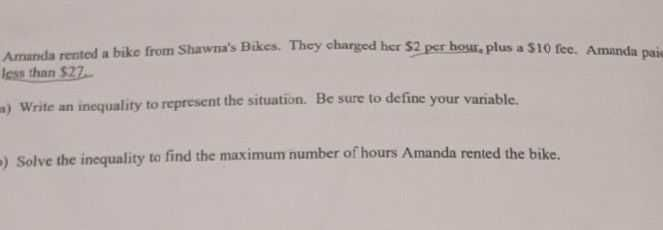### Still have math questions?

Algebra
QuestionAmanda rented a bike from Shawra's Bikes. They charged her $$52$$ per hour, plus a $$510$$ fee. Amanda paic Iess than $$\ 27$$ .

(a) Wrife an incquality to reprusent the situation. Be sure to define your variable.

(B)Solve the inequality to find the maximum number of hours Amanda rented the bike.

(a) $$2x+ 10< 27$$
(b) $$x< 8.5$$